Main Content

# Speaker Identification Using Pitch and MFCC

This example demonstrates a machine learning approach to identify people based on features extracted from recorded speech. The features used to train the classifier are the pitch of the voiced segments of the speech and the mel frequency cepstrum coefficients (MFCC). This is a closed-set speaker identification: the audio of the speaker under test is compared against all the available speaker models (a finite set) and the closest match is returned.

### Introduction

The approach used in this example for speaker identification is shown in the diagram.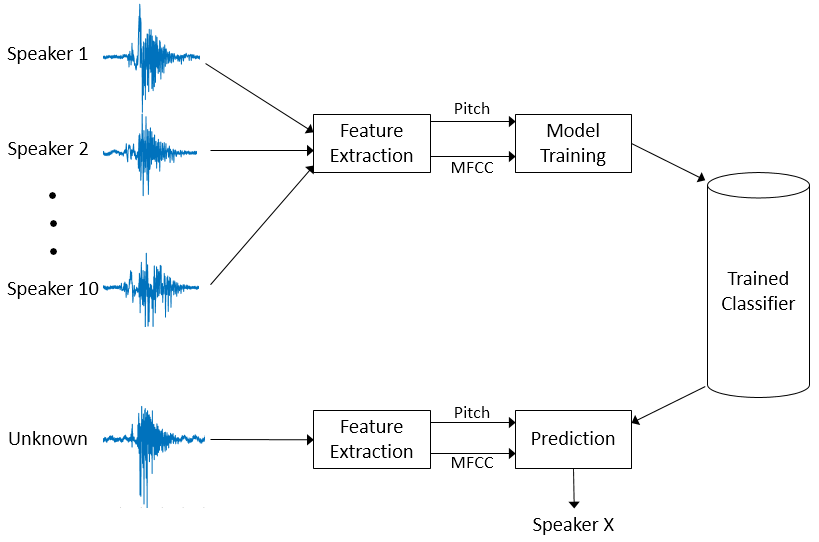Pitch and MFCC are extracted from speech signals recorded for 10 speakers. These features are used to train a K-nearest neighbor (KNN) classifier. Then, new speech signals that need to be classified go through the same feature extraction. The trained KNN classifier predicts which one of the 10 speakers is the closest match.

### Features Used for Classification

This section discusses pitch and MFCC, the two features that are used to classify speakers.

Pitch

Speech can be broadly categorized as voiced and unvoiced. In the case of voiced speech, air from the lungs is modulated by vocal cords and results in a quasi-periodic excitation. The resulting sound is dominated by a relatively low-frequency oscillation, referred to as pitch. In the case of unvoiced speech, air from the lungs passes through a constriction in the vocal tract and becomes a turbulent, noise-like excitation. In the source-filter model of speech, the excitation is referred to as the source, and the vocal tract is referred to as the filter. Characterizing the source is an important part of characterizing the speech system.

As an example of voiced and unvoiced speech, consider a time-domain representation of the word "two" (/T UW/). The consonant /T/ (unvoiced speech) looks like noise, while the vowel /UW/ (voiced speech) is characterized by a strong fundamental frequency.

```[audioIn, fs] = audioread('Counting-16-44p1-mono-15secs.wav'); twoStart = 110e3; twoStop = 135e3; audioIn = audioIn(twoStart:twoStop); timeVector = linspace((twoStart/fs),(twoStop/fs),numel(audioIn)); sound(audioIn,fs) figure plot(timeVector,audioIn) axis([(twoStart/fs) (twoStop/fs) -1 1]) ylabel('Amplitude') xlabel('Time (s)') title('Utterance - Two')```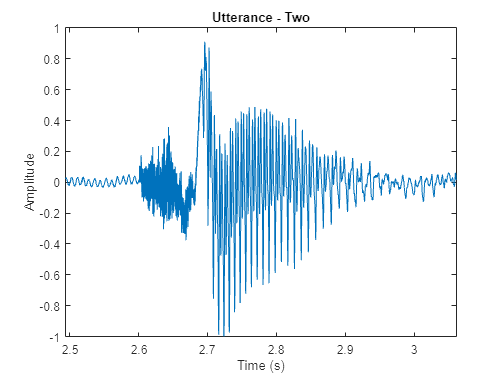A speech signal is dynamic in nature and changes over time. It is assumed that speech signals are stationary on short time scales, and their processing is done in windows of 20-40 ms. This example uses a 30 ms window with a 25 ms overlap. Use the `pitch` function to see how the pitch changes over time.

```windowLength = round(0.03*fs); overlapLength = round(0.025*fs); f0 = pitch(audioIn,fs,'WindowLength',windowLength,'OverlapLength',overlapLength,'Range',[50,250]); figure subplot(2,1,1) plot(timeVector,audioIn) axis([(110e3/fs) (135e3/fs) -1 1]) ylabel('Amplitude') xlabel('Time (s)') title('Utterance - Two') subplot(2,1,2) timeVectorPitch = linspace((twoStart/fs),(twoStop/fs),numel(f0)); plot(timeVectorPitch,f0,'*') axis([(110e3/fs) (135e3/fs) min(f0) max(f0)]) ylabel('Pitch (Hz)') xlabel('Time (s)') title('Pitch Contour')```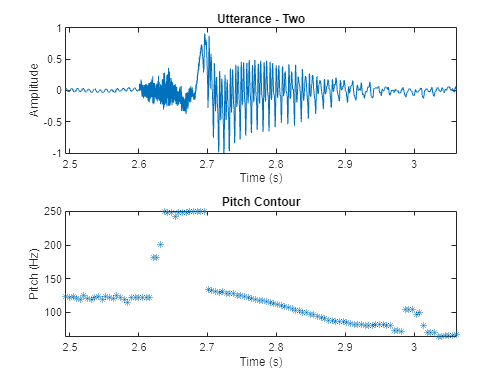The `pitch` function estimates a pitch value for every frame. However, pitch is only characteristic of a source in regions of voiced speech. The simplest method to distinguish between silence and speech is to analyze the short term power. If the power in a frame is above a given threshold, you declare the frame as speech.

```pwrThreshold = -20; [segments,~] = buffer(audioIn,windowLength,overlapLength,'nodelay'); pwr = pow2db(var(segments)); isSpeech = (pwr > pwrThreshold);```

The simplest method to distinguish between voiced and unvoiced speech is to analyze the zero crossing rate. A large number of zero crossings implies that there is no dominant low-frequency oscillation. If the zero crossing rate for a frame is below a given threshold, you declare it as voiced.

```zcrThreshold = 300; zeroLoc = (audioIn==0); crossedZero = logical([0;diff(sign(audioIn))]); crossedZero(zeroLoc) = false; [crossedZeroBuffered,~] = buffer(crossedZero,windowLength,overlapLength,'nodelay'); zcr = (sum(crossedZeroBuffered,1)*fs)/(2*windowLength); isVoiced = (zcr < zcrThreshold);```

Combine `isSpeech` and `isVoiced` to determine whether a frame contains voiced speech.

`voicedSpeech = isSpeech & isVoiced;`

Remove regions that do not correspond to voiced speech from the pitch estimate and plot.

```f0(~voicedSpeech) = NaN; figure subplot(2,1,1) plot(timeVector,audioIn) axis([(110e3/fs) (135e3/fs) -1 1]) axis tight ylabel('Amplitude') xlabel('Time (s)') title('Utterance - Two') subplot(2,1,2) plot(timeVectorPitch,f0,'*') axis([(110e3/fs) (135e3/fs) min(f0) max(f0)]) ylabel('Pitch (Hz)') xlabel('Time (s)') title('Pitch Contour')```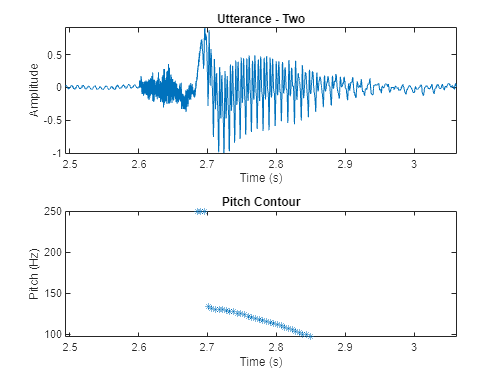Mel-Frequency Cepstrum Coefficients (MFCC)

MFCC are popular features extracted from speech signals for use in recognition tasks. In the source-filter model of speech, MFCC are understood to represent the filter (vocal tract). The frequency response of the vocal tract is relatively smooth, whereas the source of voiced speech can be modeled as an impulse train. The result is that the vocal tract can be estimated by the spectral envelope of a speech segment.

The motivating idea of MFCC is to compress information about the vocal tract (smoothed spectrum) into a small number of coefficients based on an understanding of the cochlea.

Although there is no hard standard for calculating MFCC, the basic steps are outlined by the diagram.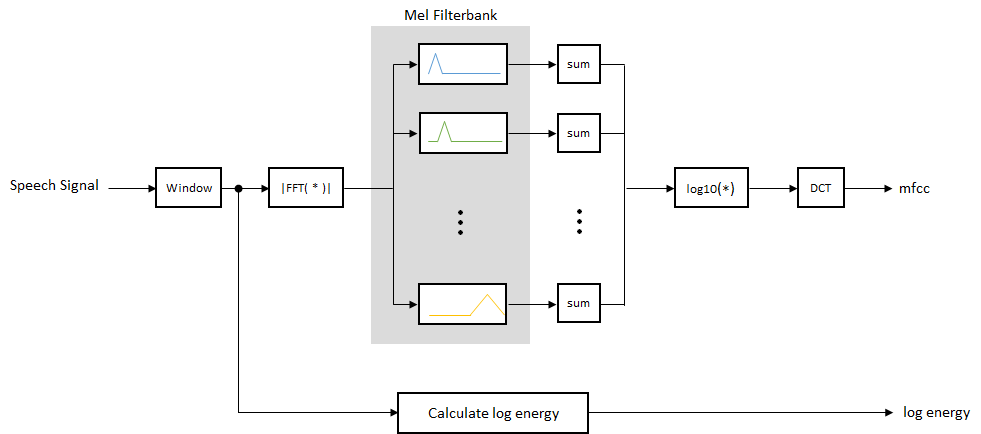The mel filterbank linearly spaces the first 10 triangular filters and logarithmically spaces the remaining filters. The individual bands are weighted for even energy. The graph represents a typical mel filterbank.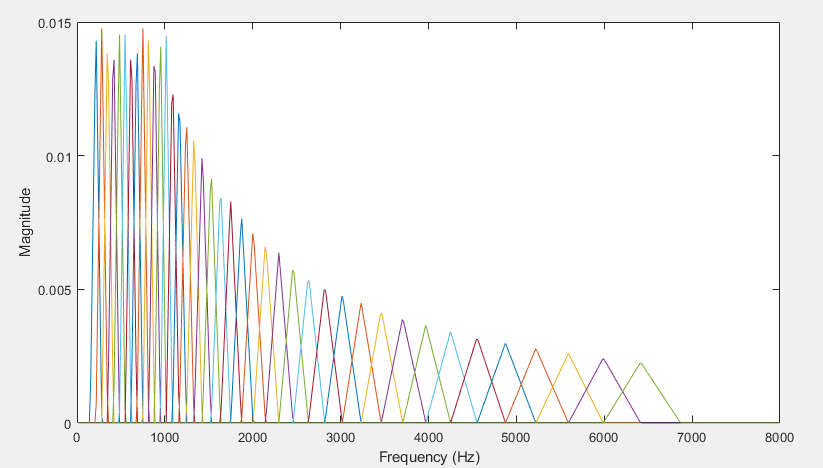This example uses `mfcc` to calculate the MFCC for every file.

### Data Set

This example uses the Census Database (also known as AN4 Database) from the CMU Robust Speech Recognition Group . The data set contains recordings of male and female subjects speaking words and numbers. The helper function in this section downloads it for you and converts the raw files to FLAC. The speech files are partitioned into subdirectories based on the labels corresponding to the speakers. If you are unable to download it, you can load a table of features from `HelperAN4TrainingFeatures.mat` and proceed directly to the Training a Classifier section. The features have been extracted from the same data set.

Download and extract the speech files for 10 speakers (5 female and 5 male) into a temporary directory using the `HelperAN4Download` function.

`dataDir = HelperAN4Download;`

Create an `audioDatastore` object to manage this database for training. The datastore allows you to collect necessary files of a file format and read them.

```ads = audioDatastore(dataDir,'IncludeSubfolders',true, ... 'FileExtensions','.flac', ... 'LabelSource','foldernames')```
```ads = audioDatastore with properties: Files: { ' ...\scrawfor\AppData\Local\Temp\an4\wav\flacData\fejs\an36-fejs-b.flac'; ' ...\scrawfor\AppData\Local\Temp\an4\wav\flacData\fejs\an37-fejs-b.flac'; ' ...\scrawfor\AppData\Local\Temp\an4\wav\flacData\fejs\an38-fejs-b.flac' ... and 122 more } Folders: { 'C:\Users\scrawfor\AppData\Local\Temp\an4\wav\flacData' } Labels: [fejs; fejs; fejs ... and 122 more categorical] AlternateFileSystemRoots: {} OutputDataType: 'double' SupportedOutputFormats: ["wav" "flac" "ogg" "mp4" "m4a"] DefaultOutputFormat: "wav" ```

The `splitEachLabel` function of `audioDatastore` splits the datastore into two or more datastores. The resulting datastores have the specified proportion of the audio files from each label. In this example, the datastore is split into two parts. 80% of the data for each label is used for training, and the remaining 20% is used for testing. The `countEachLabel` method of `audioDatastore` is used to count the number of audio files per label. In this example, the label identifies the speaker.

`[adsTrain, adsTest] = splitEachLabel(ads,0.8);`

Display the datastore and the number of speakers in the train datastore.

`adsTrain`
```adsTrain = audioDatastore with properties: Files: { ' ...\scrawfor\AppData\Local\Temp\an4\wav\flacData\fejs\an36-fejs-b.flac'; ' ...\scrawfor\AppData\Local\Temp\an4\wav\flacData\fejs\an37-fejs-b.flac'; ' ...\scrawfor\AppData\Local\Temp\an4\wav\flacData\fejs\an38-fejs-b.flac' ... and 94 more } Folders: { 'C:\Users\scrawfor\AppData\Local\Temp\an4\wav\flacData' } Labels: [fejs; fejs; fejs ... and 94 more categorical] AlternateFileSystemRoots: {} OutputDataType: 'double' SupportedOutputFormats: ["wav" "flac" "ogg" "mp4" "m4a"] DefaultOutputFormat: "wav" ```
`trainDatastoreCount = countEachLabel(adsTrain)`
```trainDatastoreCount=10×2 table Label Count _____ _____ fejs 10 fmjd 10 fsrb 10 ftmj 10 fwxs 10 mcen 10 mrcb 10 msjm 10 msjr 10 msmn 7 ```

Display the datastore and the number of speakers in the test datastore.

`adsTest`
```adsTest = audioDatastore with properties: Files: { ' ...\scrawfor\AppData\Local\Temp\an4\wav\flacData\fejs\cen6-fejs-b.flac'; ' ...\scrawfor\AppData\Local\Temp\an4\wav\flacData\fejs\cen7-fejs-b.flac'; ' ...\scrawfor\AppData\Local\Temp\an4\wav\flacData\fejs\cen8-fejs-b.flac' ... and 25 more } Folders: { 'C:\Users\scrawfor\AppData\Local\Temp\an4\wav\flacData' } Labels: [fejs; fejs; fejs ... and 25 more categorical] AlternateFileSystemRoots: {} OutputDataType: 'double' SupportedOutputFormats: ["wav" "flac" "ogg" "mp4" "m4a"] DefaultOutputFormat: "wav" ```
`testDatastoreCount = countEachLabel(adsTest)`
```testDatastoreCount=10×2 table Label Count _____ _____ fejs 3 fmjd 3 fsrb 3 ftmj 3 fwxs 2 mcen 3 mrcb 3 msjm 3 msjr 3 msmn 2 ```

To preview the content of your datastore, read a sample file and play it using your default audio device.

```[sampleTrain, dsInfo] = read(adsTrain); sound(sampleTrain,dsInfo.SampleRate)```

Reading from the train datastore pushes the read pointer so that you can iterate through the database. Reset the train datastore to return the read pointer to the start for the following feature extraction.

`reset(adsTrain)`

### Feature Extraction

Extract pitch and MFCC features from each frame that corresponds to voiced speech in the training datastore. The supporting function, isVoicedSpeech, performs the voicing detection outlined in the description of pitch feature extraction.

```fs = dsInfo.SampleRate; windowLength = round(0.03*fs); overlapLength = round(0.025*fs); features = []; labels = []; while hasdata(adsTrain) [audioIn,dsInfo] = read(adsTrain); melC = mfcc(audioIn,fs,'Window',hamming(windowLength,'periodic'),'OverlapLength',overlapLength); f0 = pitch(audioIn,fs,'WindowLength',windowLength,'OverlapLength',overlapLength); feat = [melC,f0]; voicedSpeech = isVoicedSpeech(audioIn,fs,windowLength,overlapLength); feat(~voicedSpeech,:) = []; label = repelem(dsInfo.Label,size(feat,1)); features = [features;feat]; labels = [labels,label]; end```

Pitch and MFCC are not on the same scale. This will bias the classifier. Normalize the features by subtracting the mean and dividing the standard deviation.

```M = mean(features,1); S = std(features,[],1); features = (features-M)./S;```

### Training a Classifier

Now that you have collected features for all 10 speakers, you can train a classifier based on them. In this example, you use a K-nearest neighbor (KNN) classifier. KNN is a classification technique naturally suited for multiclass classification. The hyperparameters for the nearest neighbor classifier include the number of nearest neighbors, the distance metric used to compute distance to the neighbors, and the weight of the distance metric. The hyperparameters are selected to optimize validation accuracy and performance on the test set. In this example, the number of neighbors is set to 5 and the metric for distance chosen is squared-inverse weighted Euclidean distance. For more information about the classifier, refer to `fitcknn` (Statistics and Machine Learning Toolbox).

Train the classifier and print the cross-validation accuracy. `crossval` (Statistics and Machine Learning Toolbox) and `kfoldLoss` (Statistics and Machine Learning Toolbox) are used to compute the cross-validation accuracy for the KNN classifier.

Specify all the classifier options and train the classifier.

```trainedClassifier = fitcknn( ... features, ... labels, ... 'Distance','euclidean', ... 'NumNeighbors',5, ... 'DistanceWeight','squaredinverse', ... 'Standardize',false, ... 'ClassNames',unique(labels));```

Perform cross-validation.

```k = 5; group = labels; c = cvpartition(group,'KFold',k); % 5-fold stratified cross validation partitionedModel = crossval(trainedClassifier,'CVPartition',c);```

Compute the validation accuracy.

```validationAccuracy = 1 - kfoldLoss(partitionedModel,'LossFun','ClassifError'); fprintf('\nValidation accuracy = %.2f%%\n', validationAccuracy*100);```
```Validation accuracy = 97.64% ```

Visualize the confusion chart.

```validationPredictions = kfoldPredict(partitionedModel); figure cm = confusionchart(labels,validationPredictions,'title','Validation Accuracy'); cm.ColumnSummary = 'column-normalized'; cm.RowSummary = 'row-normalized';```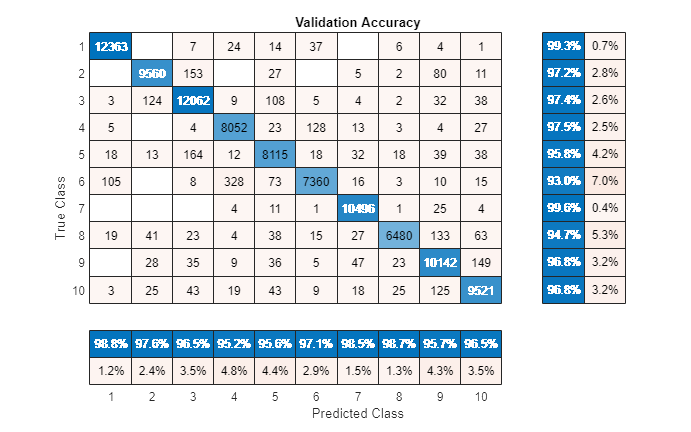You can also use the Classification Learner (Statistics and Machine Learning Toolbox) app to try out and compare various classifiers with your table of features.

### Testing the Classifier

In this section, you test the trained KNN classifier with speech signals from each of the 10 speakers to see how well it behaves with signals that were not used to train it.

Read files, extract features from the test set, and normalize them.

```features = []; labels = []; numVectorsPerFile = []; while hasdata(adsTest) [audioIn,dsInfo] = read(adsTest); melC = mfcc(audioIn,fs,'Window',hamming(windowLength,'periodic'),'OverlapLength',overlapLength); f0 = pitch(audioIn,fs,'WindowLength',windowLength,'OverlapLength',overlapLength); feat = [melC,f0]; voicedSpeech = isVoicedSpeech(audioIn,fs,windowLength,overlapLength); feat(~voicedSpeech,:) = []; numVec = size(feat,1); label = repelem(dsInfo.Label,numVec); numVectorsPerFile = [numVectorsPerFile,numVec]; features = [features;feat]; labels = [labels,label]; end features = (features-M)./S;```

Predict the label (speaker) for each frame by calling `predict` on `trainedClassifier`.

```prediction = predict(trainedClassifier,features); prediction = categorical(string(prediction));```

Visualize the confusion chart.

```figure('Units','normalized','Position',[0.4 0.4 0.4 0.4]) cm = confusionchart(labels,prediction,'title','Test Accuracy (Per Frame)'); cm.ColumnSummary = 'column-normalized'; cm.RowSummary = 'row-normalized';```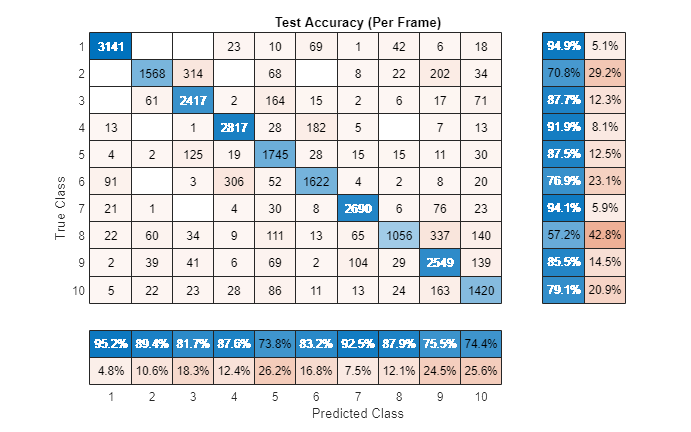For a given file, predictions are made for every frame. Determine the mode of predictions for each file and then plot the confusion chart.

```r2 = prediction(1:numel(adsTest.Files)); idx = 1; for ii = 1:numel(adsTest.Files) r2(ii) = mode(prediction(idx:idx+numVectorsPerFile(ii)-1)); idx = idx + numVectorsPerFile(ii); end figure('Units','normalized','Position',[0.4 0.4 0.4 0.4]) cm = confusionchart(adsTest.Labels,r2,'title','Test Accuracy (Per File)'); cm.ColumnSummary = 'column-normalized'; cm.RowSummary = 'row-normalized';```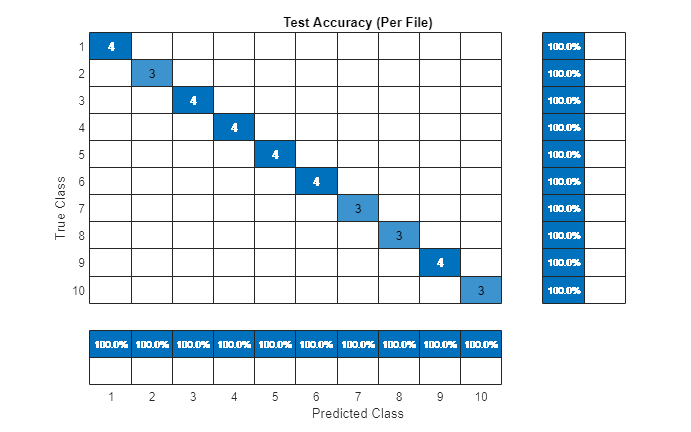The predicted speakers match the expected speakers for all files under test.

The experiment was repeated using an internally developed dataset. The dataset consists of 20 speakers with each speaker speaking multiple sentences from the Harvard sentence list . For 20 speakers, the validation accuracy 89%.

### Supporting Functions

```function voicedSpeech = isVoicedSpeech(x,fs,windowLength,overlapLength) pwrThreshold = -40; [segments,~] = buffer(x,windowLength,overlapLength,'nodelay'); pwr = pow2db(var(segments)); isSpeech = (pwr > pwrThreshold); zcrThreshold = 1000; zeroLoc = (x==0); crossedZero = logical([0;diff(sign(x))]); crossedZero(zeroLoc) = false; [crossedZeroBuffered,~] = buffer(crossedZero,windowLength,overlapLength,'nodelay'); zcr = (sum(crossedZeroBuffered,1)*fs)/(2*windowLength); isVoiced = (zcr < zcrThreshold); voicedSpeech = isSpeech & isVoiced; end```

### References

 "CMU Sphinx Group - Audio Databases." Accessed December 19, 2019. http://www.speech.cs.cmu.edu/databases/an4/.

 "Harvard Sentences." Wikipedia, 27 Aug. 2019. Wikipedia, https://en.wikipedia.org/w/index.php?title=Harvard_sentences&oldid=912785385.

## SupportGet trial now